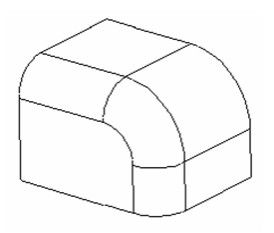Mitering the vertices produces rounded edges that meet at an edge at the corners. This example will use the following model.1. Make sure Round vertex is disabled.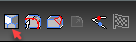1. Select the object whose edges are to be rounded. Each edge is marked with a blue square.

2. Set the Start Radius radius in the Inspector Bar, and select the edges.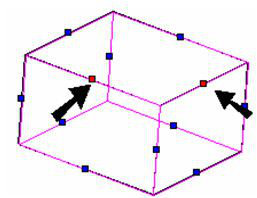1. The edges is rounded, and meet at a sharp corner.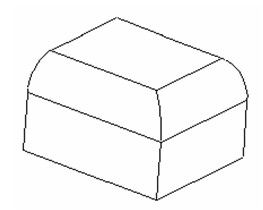1. To round another edge, select the object again and select the next edge. Make sure Pick smooth sequence is not selected.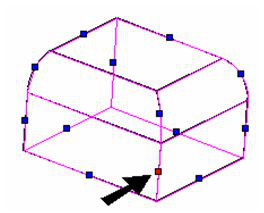This is the result - the intersection of all three corners has sharp edges.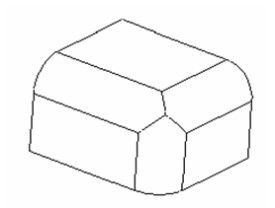# Pick Smooth Sequence

Adjacent edges are smoothly connected if they are connected by an arc or rounded vertex. When working with Round vertex, smoothly connected edges are automatically selected. But this is not the case when Round vertex is turned off.

1. Start with a box with one filleted edge, using a large radius.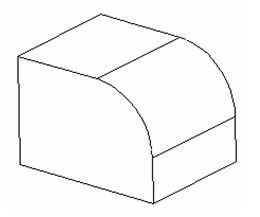1. Activate Fillet without Round vertex, and fillet one edge, using a smaller radius.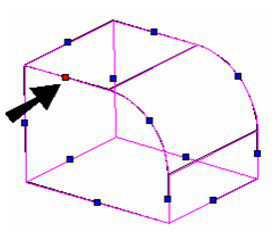This is the result - only the selected edge was rounded.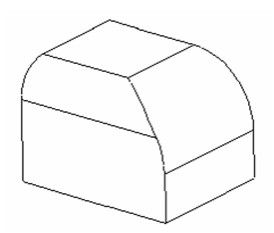1. Undo, and this time select Pick smooth sequence.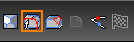1. This time when you select one of these edges for filleting, all edges in the smooth chain are selected.This is the result - all edges in the chain are rounded.